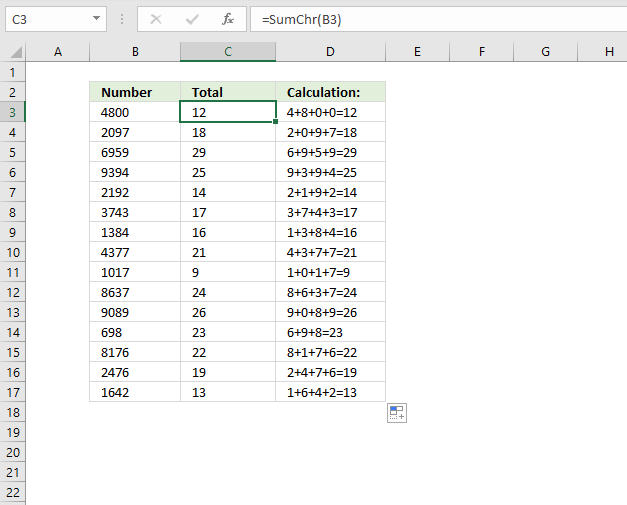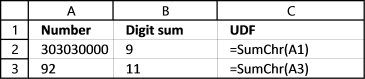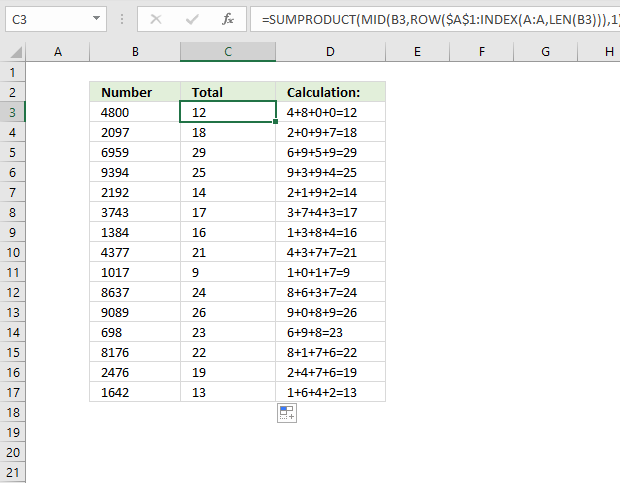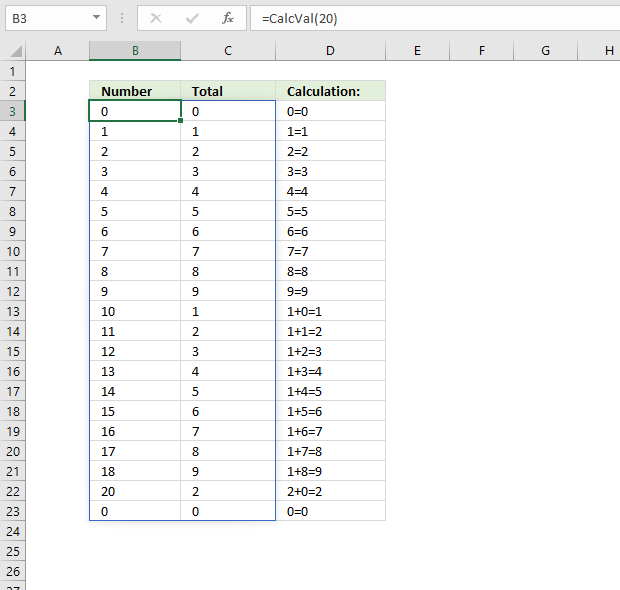Author: Oscar Cronquist Article last updated on June 07, 2021I got a question a while ago about permutations, in essence how to find every permutation between 0 and 9 000 000 000 with a condition, if the sum of all digits in a number is smaller or equal to 9.

You can read about the difference between combinations and permutations here: Return all combinations but in short, the order is important for permutations and not important for combinations.

This number 303 030 000 is equal to 9 if you sum every digit, 3+0+3+0+3+0+0+0+0 = 9. 92 is above 9, 9+2 = 11 and is not a number we are looking for.

## 1. Sum all digits in a number - User Defined Function (UDF)The following user-defined function named  SumChr adds all digits in a number and returns the total. The image above demonstrates the SumChr function in column B.

For example, number 92 in cell A3 contains two digits, 9 and 2. The total is 9 + 2 equals 11. The SumChr function returns 11 in cell B3.

```'Name User Defined Function and specify parameters
Function SumChr(nmbr As Long)

'Dimension variables and declare data types
Dim sm As Long

'For ... Next statement
For i = 1 To Len(CStr(nmbr))

'Add number to total and save the total
sm = sm + Val(Mid(nmbr, i, 1))

'Continue with next digit
Next i

SumChr = sm
End Function
```

## 2. Sum all digits in a number (formula)Formula in cell C3:

=SUMPRODUCT(MID(B3, ROW(\$A\$1:INDEX(A:A, LEN(B3))), 1)*1)

Excel 365 dynamic array formula in cell C3:

=SUM(MID(B3, SEQUENCE(LEN(B3)), 1)*1)

## 3. Generate permutations based on a condition (slow UDF)It is easy to iterate through every number between 0 and for example 10 000 000 and check if the digit sum is smaller or equal to 9. The UDF below does that.

The picture above shows all numbers between 0 and 20 where the digit sum is smaller or equal to 9, the CalcVal function above made this list.

Number 19 is missing, 1+9 = 10. 10 is bigger than 9. Column B contains the digit sum, except cell B1. It has the time it took to calculate the list. In this case, it took almost no time at all, and excel rounded it to 0 (zero).

```'Name User Defined Function and specify parameters
Function CalcVal(k As Double)

'Dimension variables and declare data types
Dim i As Long, j() As Double, a As Double

'Redimension array variable j
ReDim j(0 To 60000, 1)

'Save timer value to variable a
a = Timer

'Save 0 (zero) to variable l
l = 0

'For ... Next statement
'Iterate from 0 (zero) to number stored in variable k
For i = 0 To k

'Calculate total using UDF SumChr based on number stored in variable i
m = SumChr(i)

Check if variable m is equal to 9
If m &lt;= 9 Then

'Save value stored in variable i to array variable j
j(l, 0) = i

'Save value stored in variable m to array variable j
j(l, 1) = m

'Add one to value stored in variable l and save to variable l
l = l + 1
End If
Next i

'Calculate time needed calculate result and save to array variable j
j(0, 1) = Timer - a

'Return array variable j to worksheet
CalcVal = j()
End Function
```

## 4. Generate permutations based on a condition (faster UDF)

The problem is if you try to do the same with a really large range of numbers, it will take a very long time to calculate for a desktop pc. This UDF shortens that time considerably.

```'Name User Defined Function and specify parameters
Function CalcVal1(k As Single)

'Dimension variables and declare data types
Dim i As Single, j() As Double, a As Double
Dim l As Single, m As Single

'Save time value to variable a
a = Timer

'Redimension array variable j
ReDim j(0 To 2000000, 1)

'Save -1 to variable i and 0 (zero) to variable m
i = -1: m = 0

'Do Until ... Loop
'Keep iterating until number in variable k is maller than or equal to number in variable i
Do Until k &lt;= i

'If ... Then .. ElseIf .. Endif statement
'Check if total is equal to or less than 9
If SumChr(i) &lt;= 9 Then

'Convert variable i to string and then count characters in string, save number of characters to variable p
p = Len(CStr(i))

'For ... Next statement
'Go backwards from number of characters in variable i to 1
For l = Len(CStr(i)) To 1 Step -1

'If ... Then ... ElseIf ... Esle ... End If statement
'Check if characters is not equal to 0 (zero) and not equal to 9
If Mid(i, l, 1) <> 0 And Mid(i, l, 1) <> 9 Then

'Replace character in string
i = Val(ReplaceChrInStr(i, l, 0))
i = Val(ReplaceChrInStr(i, l - 1, Mid(i, l - 1, 1) + 1))
Exit For
ElseIf Mid(i, l, 1) = 9 Then
i = i + Application.WorksheetFunction.Power(10, Len(CStr(i)) - 1)
Exit For
End If
Next l
Else
i = i + 1
End If
If i &lt; k Then
j(m, 0) = i
Else
j(m, 0) = ""
End If
j(m, 1) = SumChr(i)
m = m + 1
Loop
j(0, 1) = Timer - a
CalcVal1 = j
End Function
```
```Function ReplaceChrInStr(tmp As String, pos As Single, chr As String)
Dim str As String, i As Single
For i = 1 To Len(tmp)
If i = pos Then
str = str & chr
Else
str = str & Mid(tmp, i, 1)
End If
Next i
ReplaceChrInStr = str
End Function
```

The UDF basically examines each digit in a number and if the sum is 9 these things happens:

• If digit is not equal to 9 and zero, make that digit a 0 and add 1 to the next digit.
• If digit is equal to 9, add 1,10, 100... depending on the position (n) the digit has in the number. Example, digit 9 in number 90 is the second digit counting from right to left, n=2. 10^(2-1) is 10. 90+10 = 100

If the digit sum is not equal to 9 the udf adds 1 to the current number and the loop continues until the entire range has been created.

## 5. Comparing custom functions

Now lets compare the run times of the two udfs CalcVal and CalcVal1.For smaller ranges like 0 - 9000 CalcVal is faster, somewhere around perhaps 5000 CalcVal1 seems to take the lead.

I am using an old laptop with a dual-core intel i3 cpu, I am getting 29% utilization. I don't know why, it seems that only one core is utilized by excel. In any case, the numbers indicate that the CalcVal1 function is much faster.

## 6. Where to put the code?

1. Press Alt + F11 to open the Visual Basic Editor (VBE).
2. Press with mouse on "Insert" on the top menu.
3. Press with mouse on "Module".
4. Copy above UDFs and paste to the code window.